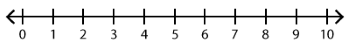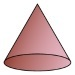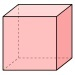##### Notes

Daily Math Practice provides a week's worth of problems ideal for morning work or review. Week 20 reviews standards from previous weeks and supports Common Core Mathematics Standards K.OA.A.1, K.OA.A.2, & K.OA.A.3.

##### Print Instructions

NOTE: Only your test content will print.
To preview this test, click on the File menu and select Print Preview.

See our guide on How To Change Browser Print Settings to customize headers and footers before printing.

# Kindergarten - Daily Math Practice - Week 20 (Kindergarten)

Print Test (Only the test content will print)
 DAILY MATH PRACTICE - WEEK 20 DAY 1 DAY 2 Subtract. Use the number line.4 - 1 = Subtract. Use the number line.3 - 1 = There are 5 birds on a branch. Then, 1 bird flies away. How many birds are left on the branch? Draw a picture:Answer:birds Lilly needs 6 cups to set the table. She only has 4 cups. How many cups does Lilly need?Write a subtraction sentence:Answer:cups Circle the addition sentence for the model.1 + 4 = 52 + 3 = 5 Circle the addition sentence for the model.4 + 1 = 53 + 2 = 5 Circle the smaller number.10 OR 7 Circle the cube.DAILY MATH PRACTICE - WEEK 20 DAY 3 DAY 4 Subtract. Use the number line.3 - 3 = Subtract. Use the number line.9 - 3 = There are 4 frogs on a log. Then, 1 frog hops into the water. How many frogs are left on the log?Draw a picture:Answer:frogs Peter has 8 balloons. He gives 4 balloons to his friends. How many balloons does Peter have left?Write a subtraction sentence:Answer:balloons Circle the addition sentence for the model.2 + 3 = 51 + 4 = 5 Circle the addition sentence for the model.5 + 0 = 54 + 1 = 5 How many acorns? Circle the number.6 7 8 Write the missing number.18 = 1 ten +onesYou need to be a HelpTeaching.com member to access free printables.